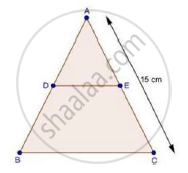Share

Books Shortlist
Your shortlist is empty

# Solution for In δAbc, D and E Are Points on the Sides Ab and Ac Respectively Such that De || Bc If "Ad"/"Db"=3/4 And Ac = 15 Cm, Find Ae - CBSE Class 10 - Mathematics

ConceptBasic Proportionality Theorem Or Thales Theorem

#### Question

In ΔABC, D and E are points on the sides AB and AC respectively such that DE || BC

If "AD"/"DB"=3/4 and AC = 15 cm, find AE

#### SolutionWe have,

"AD"/"DB"=3/4 and DE || BC

Therefore, by basic proportionality theorem, we have

"AD"/"DB"="AE"/"EC"

Adding 1 on both sides, we get

"AD"/"DB"+1="AE"/"EC"+1

3/4+1=("AE"+"EC")/"EC"

rArr(3+4)/4="AC"/"EC"               [∵ AE + EC = AC]

rArr7/4=15/"EC"

rArr"EC"=(15xx4)/7

rArr"EC"=60/7

Now, AE + EC = AC

rArr"AE"+60/7=15

rArr"AE"=15-60/7

=(105-60)/7

=45/7

= 6.43 cm

∴ AE = 6.43 cm

Is there an error in this question or solution?

#### Video TutorialsVIEW ALL 

Solution for question: In δAbc, D and E Are Points on the Sides Ab and Ac Respectively Such that De || Bc If "Ad"/"Db"=3/4 And Ac = 15 Cm, Find Ae concept: Basic Proportionality Theorem Or Thales Theorem. For the course CBSE
S